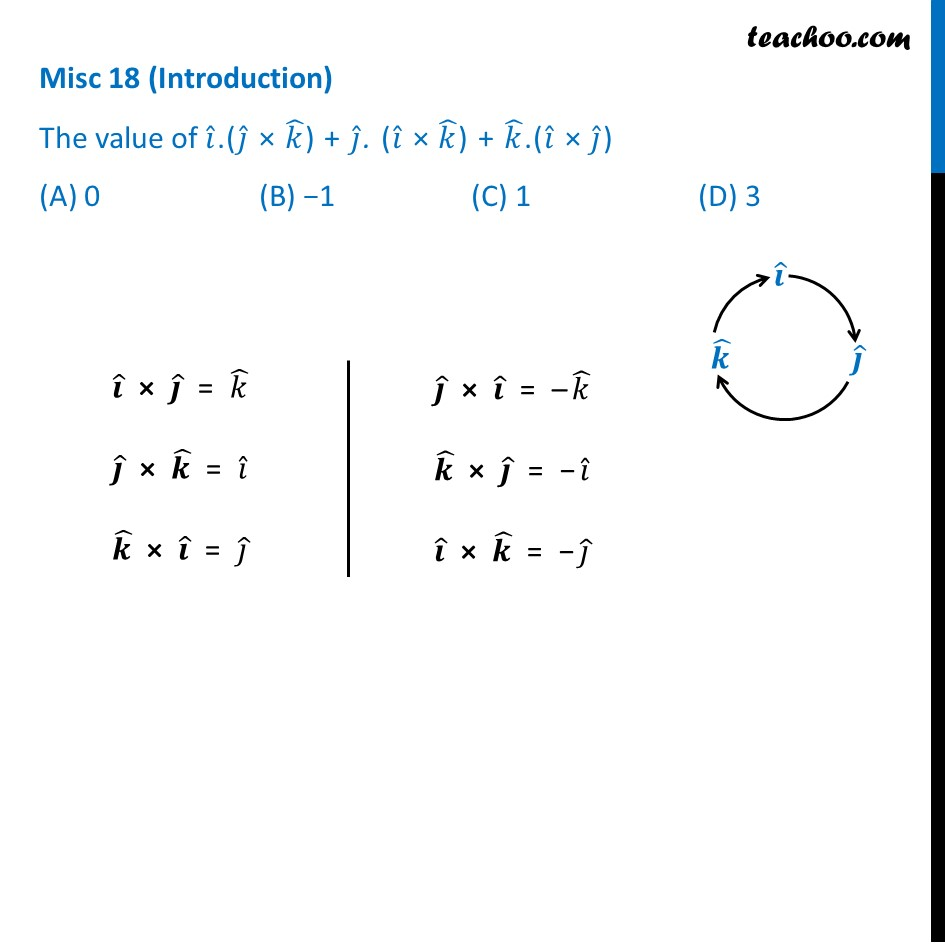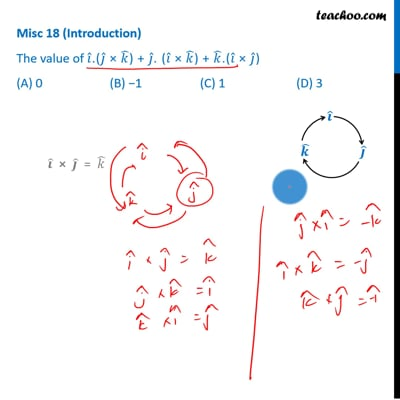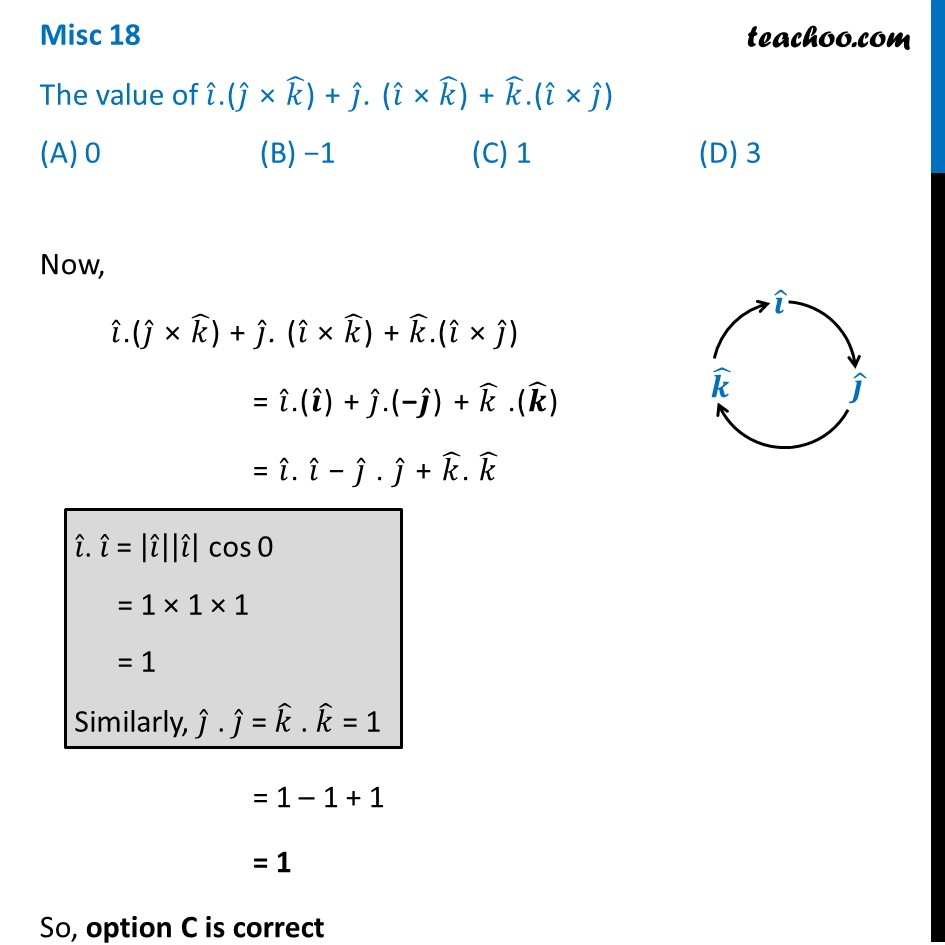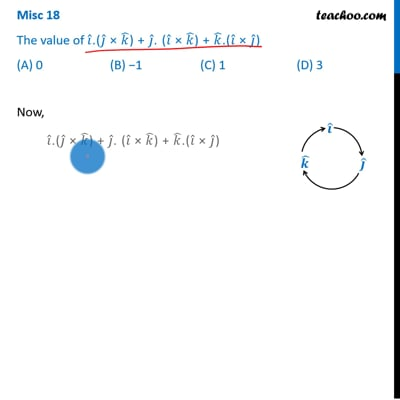Vector product - Solving

Chapter 10 Class 12 Vector Algebra
Concept wiseThis video is only available for Teachoo black usersThis video is only available for Teachoo black users

Introducing your new favourite teacher - Teachoo Black, at only ₹83 per month

### Transcript

Misc 18 (Introduction) The value of 𝑖 ̂.(𝑗 ̂ × 𝑘 ̂) + 𝑗 ̂. (𝑖 ̂ × 𝑘 ̂) + 𝑘 ̂.(𝑖 ̂ × 𝑗 ̂) (A) 0 (B) −1 (C) 1 (D) 3 𝒊 ̂ × 𝒋 ̂ = 𝑘 ̂ 𝒋 ̂ × 𝒌 ̂ = 𝑖 ̂ 𝒌 ̂ × 𝒊 ̂ = 𝑗 ̂ 𝒋 ̂ × 𝒊 ̂ = –𝑘 ̂ 𝒌 ̂ × 𝒋 ̂ = −𝑖 ̂ 𝒊 ̂ × 𝒌 ̂ = −𝑗 ̂ Misc 18 The value of 𝑖 ̂.(𝑗 ̂ × 𝑘 ̂) + 𝑗 ̂. (𝑖 ̂ × 𝑘 ̂) + 𝑘 ̂.(𝑖 ̂ × 𝑗 ̂) (A) 0 (B) −1 (C) 1 (D) 3 Now, 𝑖 ̂.(𝑗 ̂ × 𝑘 ̂) + 𝑗 ̂. (𝑖 ̂ × 𝑘 ̂) + 𝑘 ̂.(𝑖 ̂ × 𝑗 ̂) = 𝑖 ̂.(𝒊 ̂) + 𝑗 ̂.(−𝒋 ̂) + 𝑘 ̂ .(𝒌 ̂) = 𝑖 ̂. 𝑖 ̂ − 𝑗 ̂ . 𝑗 ̂ + 𝑘 ̂. 𝑘 ̂ = 1 – 1 + 1 = 1 So, option C is correct 𝑖 ̂. 𝑖 ̂ = |𝑖 ̂ ||𝑖 ̂ | cos 0 = 1 × 1 × 1 = 1 Similarly, 𝑗 ̂ . 𝑗 ̂ = 𝑘 ̂ . 𝑘 ̂ = 1